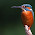## 27 December 2007

### Proof that 4 equals 3

Begin with a simple algebraic equation:
a + b = c

Now, replace each letter with 4 of that letter minus 3 of that letter:
4a - 3a + 4b - 3b = 4c - 3c

Rearrange, so that the 4s are on the left and the 3s on the right
4a + 4b - 4c = 3a + 3b - 3c

Simplify by factoring:
4(a + b - c) = 3(a + b - c)

Then divide each side by a+b-c:
4 = 3

There is, of course, a fallacy in this process. I invite someone to point out the defect with an appropriate comment in the "comments" section.

1.a(4-3) + b(4-3) = c(4-3) or
(4a-3a) + (4b-3b) = (4c-3c)
in my view, each expression in parenthesis must be evaluated before the equation can be rearranged or further modified.

2.Actually, the mathematical fallacy occurs in the final step. Since the initial equation was set up as a+b=c, that means that a+b-c is equal to zero. The last step involves dividing the equation by a+b-c. It is improper/"illegal" (and impossible) to divide anything by zero, so anything that derives from that step is fantasy (including the conclusion that 4=3).

3.i partly agree with minnesotastan;however, i would simply say there is no "illegal" division as zero divided by zero is merely redundant not exactly improper. I do love the post though, thanks for the fun!

4.actually a 0 divide by 0 is an illegal step.

simply because 0 cannot be a denominator.

5.responding to ted baker...

you do not need the parenthesis, because you are - and + like terms. it would be redundant to put the parenthesis there, and without them you could still solve this. but actually i have a different way of solving 3=4 showed to me by a mathematics teacher awhile back.

6.It's not "illegal", because that term implies going against legislative instrument, of which none exist surrounding mathematics in any nation-state on this Earth.

"Improper" is a better term. Though "evil" might be apt, as we all know what happens when you divide by zero!

BOOM!

1.7."Illegal" is defined as "contrary to, or forbidden by, law." Nature has "laws," and Einstein and others have referred to the "laws of mathematics." So, if one breaks those rules, wouldn't that transgression be "illegal?"

Ba-da-boom!

1.8.Actually, division by zero is not so much illegal as it is undefined, and it cannot be defined without contradiction. Why? Because if the expression a/0 = b were meaningful for any numbers a and b, then it would be true that a = 0*b (this is the exact meaning of the division, that is, the interpretation of the sentence y/x = z is that y = x*z). In any case, if a/0 = b then a = 0*b and therefore a = 0. You might then believe that division by zero is definable if the numerator is also zero. but that is not true either because to say that 0/0=b is to say that 0 = 0*b, which is true for all b. One cannot select a single number to be the quantity 0/0, so it cannot be defined.

9.Thank you, Matt. That's the first time I've seen it explained in that fashion. Makes perfect sense.

10.anything to the zero EXPONENT equals one, maybe thats what you're referencing.

11.(a+b-c)=0, so dividing by it is no bueno.